library: libGraf#include "TLatex.h"

# TLatex

class description - source file - inheritance tree (.pdf)

## class TLatex : public TText, public TAttLine

Inheritance Chart:
 TObject
<-
TNamed
 TAttText
<-
TText
 TAttLine
<-
TLatex
```
protected:
FormSize Anal1(TextSpec_t spec, const Char_t* t, Int_t length)
FormSize Analyse(Double_t x, Double_t y, TextSpec_t spec, const Char_t* t, Int_t length)
Int_t CheckLatexSyntax(TString& text)
void DrawCircle(Double_t x1, Double_t y1, Double_t r, TextSpec_t spec)
void DrawLine(Double_t x1, Double_t y1, Double_t x2, Double_t y2, TextSpec_t spec)
void DrawParenthesis(Double_t x1, Double_t y1, Double_t r1, Double_t r2, Double_t phimin, Double_t phimax, TextSpec_t spec)
FormSize FirstParse(Double_t angle, Double_t size, const Char_t* text)
void Savefs(FormSize* fs)

public:
TLatex()
TLatex(Double_t x, Double_t y, const char* text)
TLatex(const TLatex& text)
virtual ~TLatex()
static TClass* Class()
virtual void Copy(TObject& text) const
TLatex* DrawLatex(Double_t x, Double_t y, const char* text)
virtual void GetBoundingBox(UInt_t& w, UInt_t& h)
Double_t GetHeight() const
Double_t GetXsize()
Double_t GetYsize()
virtual TClass* IsA() const
virtual void Paint(Option_t* option)
virtual void PaintLatex(Double_t x, Double_t y, Double_t angle, Double_t size, const char* text)
virtual void SavePrimitive(ofstream& out, Option_t* option)
virtual void SetIndiceSize(Double_t factorSize)
virtual void SetLimitIndiceSize(Int_t limitFactorSize)
virtual void ShowMembers(TMemberInspector& insp, char* parent)
virtual void Streamer(TBuffer& b)
void StreamerNVirtual(TBuffer& b)
```

### Data Members

```
protected:
Double_t fFactorSize       !Relative size of subscripts and superscripts
Double_t fFactorPos        !Relative position of subscripts and superscripts
Int_t fLimitFactorSize  lower bound for subscripts/superscripts size
const Char_t* fError            !error code
Bool_t fShow             !is true during the second pass (Painting)
FormSize_t* fTabSize          !array of values for the different zones
Double_t fOriginSize       Font size of the starting font
Int_t fTabMax           !Maximum allocation for array fTabSize;
Int_t fPos              !Current position in array fTabSize;

public:
static const enum TLatex:: kTextNDC
```

## Class Description

```   TLatex : to draw Mathematical Formula

This class has been implemented by ```
Nicolas Brun
```.
========================================================

TLatex's purpose is to write mathematical equations
The syntax is very similar to the Latex one :

** Subscripts and Superscripts
------------------------------
Subscripts and superscripts are made with the _ and ^ commands.  These commands
can be combined to make complicated subscript and superscript expressions.
You may choose how to display subscripts and superscripts using the 2 functions
SetIndiceSize(Double_t) and SetLimitIndiceSize(Int_t).
```
/*p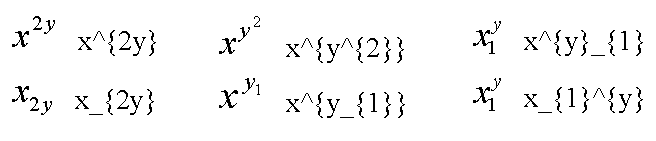*/
```
** Fractions
------------
Fractions denoted by the / symbol are made in the obvious way.
The #frac command is used for large fractions in displayed formula; it has
two arguments: the numerator and the denominator.
```
/*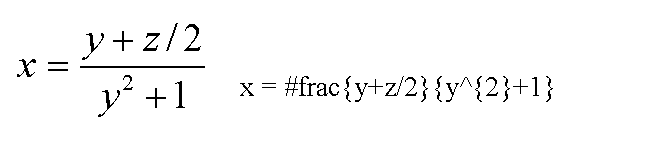*/
```
** splitting a line in two lines
--------------------------------
A text can be split in two lines via the command #splitline
For example #splitline{"21 April 2003}{14:02:30}

** Roots
--------
The #sqrt command produces the square root of its argument; it has an optional
first argument for other roots.
ex: #sqrt{10}  #sqrt{10}

** Mathematical Symbols
-----------------------
TLatex can make dozens of special mathematical symbols. A few of them, such as
+ and > , are produced by typing the corresponding keyboard character.  Others
are obtained with the commands in the following table :
```
/*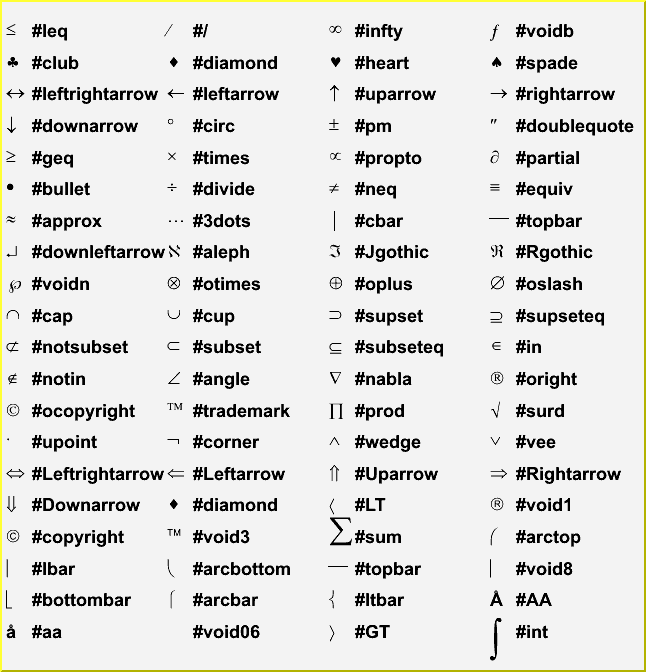*/
```    #Box draw a square

** Delimiters
-------------
You can produce 4 kinds of proportional delimiters.
#[]{....} or "a la" Latex #left[.....#right] : big square brackets
#{}{....} or              #left{.....#right} : big curly brackets
#||{....} or              #left|.....#right| : big absolute value symbol
#(){....} or              #left(.....#right) : big parenthesis

** Greek Letters
----------------
The command to produce a lowercase Greek letter is obtained by adding a # to
the name of the letter. For an uppercase Greek letter, just capitalize the first
letter of the command name. Some letter have two representations. The name of the
second one (the "variation") starts with "var".
#alpha #beta #chi #delta #varepsilon #phi #gamma #eta #iota #varphi #kappa #lambda
#mu #nu #omicron #pi #theta #rho #sigma #tau #upsilon #varomega #omega #xi #psi #zeta
#Alpha #Beta #Chi #Delta #Epsilon #Phi #Gamma #Eta #Iota #vartheta
#Kappa #Lambda #Mu #Nu #Omicron #Pi #Theta #Rho #Sigma #Tau
#Upsilon #varsigma #Omega #Xi #Psi #Zeta #varUpsilon #epsilon
```
/*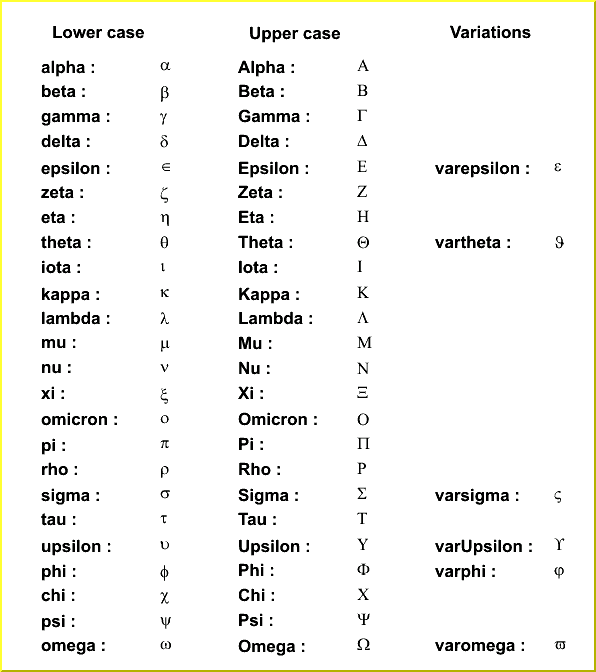*/
```
** Putting One Thing Above Another
----------------------------------
Symbols in a formula are sometimes placed on above another. TLatex provides
special commands for doing this.

** Accents
----------
#hat{a} = hat
#check  = inversed hat
#acute  = acute
#grave  = agrave
#dot    = derivative
#ddot   = double derivative
#tilde  = tilde

#slash special sign. Draw a slash on top of the text between brackets
for example #slash{E}_{T}  generates "Missing ET"

```
/*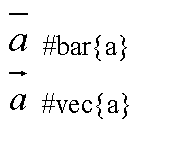*/
```   #dot  #ddot  #hat  #check  #acute  #grave  #tilde

** Changing Style in Math Mode
------------------------------
You can change the font and the text color at any moment using :
#font[font-number]{...} and #color[color-number]{...}

** Example1
-----------
The following macro (tutorials/latex.C) produces the following picture:
{
gROOT->Reset();
TCanvas c1("c1","Latex",600,700);
TLatex l;
l.SetTextAlign(12);
l.SetTextSize(0.04);
l.DrawLatex(0.1,0.8,"1)   C(x) = d #sqrt{#frac{2}{#lambdaD}}  #int^{x}_{0}cos(#frac{#pi}{2}t^{2})dt");
l.DrawLatex(0.1,0.6,"2)   C(x) = d #sqrt{#frac{2}{#lambdaD}}  #int^{x}cos(#frac{#pi}{2}t^{2})dt");
l.DrawLatex(0.1,0.4,"3)   R = |A|^{2} = #frac{1}{2}(#[]{#frac{1}{2}+C(V)}^{2}+#[]{#frac{1}{2}+S(V)}^{2})");
l.DrawLatex(0.1,0.2,"4)   F(t) = #sum_{i=-#infty}^{#infty}A(i)cos#[]{#frac{i}{t+i}}");
}
```
/*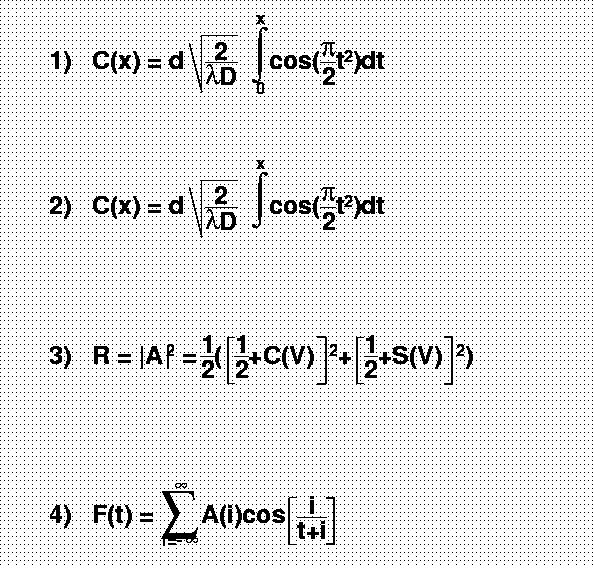*/
```
** Example2
-----------
The following macro (tutorials/latex2.C) produces the following picture:
{
gROOT->Reset();
TCanvas c1("c1","Latex",600,700);
TLatex l;
l.SetTextAlign(23);
l.SetTextSize(0.1);
l.DrawLatex(0.5,0.95,"e^{+}e^{-}#rightarrowZ^{0}#rightarrowI#bar{I}, q#bar{q}");
l.DrawLatex(0.5,0.75,"|#vec{a}#bullet#vec{b}|=#Sigmaa^{i}_{jk}+b^{bj}_{i}");
l.DrawLatex(0.5,0.5,"i(#partial_{#mu}#bar{#psi}#gamma^{#mu}+m#bar{#psi}=0#Leftrightarrow(#Box+m^{2})#psi=0");
l.DrawLatex(0.5,0.3,"L_{em}=eJ^{#mu}_{em}A_{#mu} , J^{#mu}_{em}=#bar{I}#gamma_{#mu}I , M^{j}_{i}=#SigmaA_{#alpha}#tau^{#alphaj}_{i}");
}
```
/*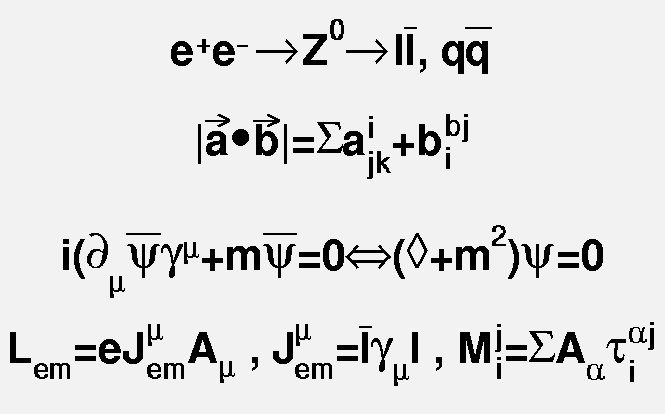*/
```
** Example3
-----------
The following macro (tutorials/latex3.C) produces the following picture:
{
gROOT->Reset();
TCanvas c1("c1");
TPaveText pt(.1,.5,.9,.9);
pt.AddText("#frac{2s}{#pi#alpha^{2}}  #frac{d#sigma}{dcos#theta} (e^{+}e^{-} #rightarrow f#bar{f} ) = ");
pt.AddText("#left| #frac{1}{1 - #Delta#alpha} #right|^{2} (1+cos^{2}#theta");
pt.AddText("+ 4 Re #left{ #frac{2}{1 - #Delta#alpha} #chi(s) #[]{#hat{g}_{#nu}^{e}#hat{g}_{#nu}^{f}
(1 + cos^{2}#theta) + 2 #hat{g}_{a}^{e}#hat{g}_{a}^{f} cos#theta) } #right}");
pt.SetLabel("Born equation");
pt.Draw();
}
```
/*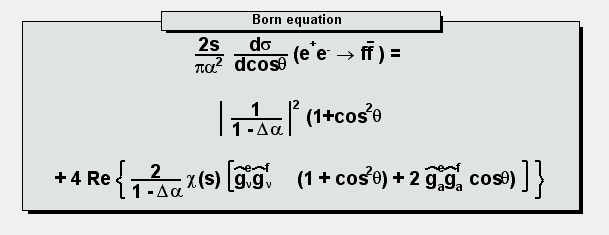*/
```
** Alignment rules
------------------
The TText alignment rules apply to the TLatex objects with one exception
concerning the vertical alignment:
If the vertical alignment = 1 , subscripts are not taken into account
if the vertical alignment = 0 , the text is aligned to the box surrounding
the full text with sub and superscripts
This is illustrated in the following example:

{
gROOT->Reset();
TCanvas c1("c1","c1",600,500);
c1.SetGrid();
c1.DrawFrame(0,0,1,1);
const char *longstring = "K_{S}... K^{*0}... #frac{2s}{#pi#alpha^{2}}
#frac{d#sigma}{dcos#theta} (e^{+}e^{-} #rightarrow f#bar{f} ) =
#left| #frac{1}{1 - #Delta#alpha} #right|^{2} (1+cos^{2}#theta)";

TLatex latex;
latex.SetTextSize(0.033);
latex.SetTextAlign(13);  //align at top
latex.DrawLatex(.2,.9,"K_{S}");
latex.DrawLatex(.3,.9,"K^{*0}");
latex.DrawLatex(.2,.8,longstring);

latex.SetTextAlign(12);  //centered
latex.DrawLatex(.2,.6,"K_{S}");
latex.DrawLatex(.3,.6,"K^{*0}");
latex.DrawLatex(.2,.5,longstring);

latex.SetTextAlign(11);  //default bottom alignment
latex.DrawLatex(.2,.4,"K_{S}");
latex.DrawLatex(.3,.4,"K^{*0}");
latex.DrawLatex(.2,.3,longstring);

latex.SetTextAlign(10);  //special bottom alignment
latex.DrawLatex(.2,.2,"K_{S}");
latex.DrawLatex(.3,.2,"K^{*0}");
latex.DrawLatex(.2,.1,longstring);

latex.SetTextAlign(12);
latex->SetTextFont(72);
latex->DrawLatex(.1,.80,"13");
latex->DrawLatex(.1,.55,"12");
latex->DrawLatex(.1,.35,"11");
latex->DrawLatex(.1,.18,"10");
}
```
/*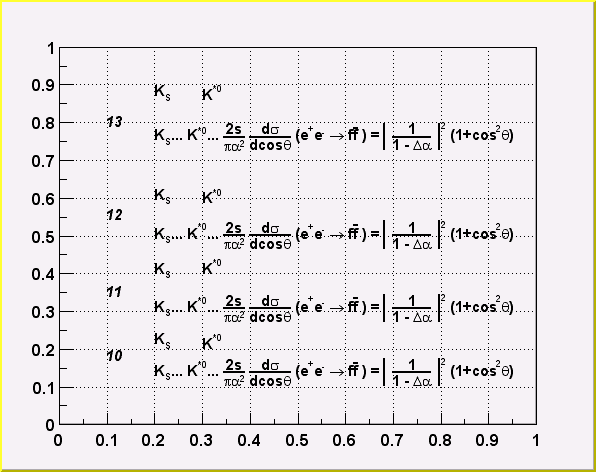*/
```______________________________________________________________________________
```

TLatex()
``` default constructor
```

TLatex(Double_t x, Double_t y, const char *text) :TText(x,y,text)
``` normal constructor
```

~TLatex()

TLatex(const TLatex &text) : TText(text), TAttLine(text)

void Copy(TObject &obj) const
```*-*-*-*-*-*-*-*-*-*-*Copy this TLatex object to another TLatex*-*-*-*-*-*-*
*-*                  =========================================
```

FormSize Anal1(TextSpec_t spec, const Char_t* t, Int_t length)

FormSize Analyse(Double_t x, Double_t y, TextSpec_t spec, const Char_t* t, Int_t length)
```  Analyse and paint the TLatex formula

It is called twice : first for calculating the size of
each portion of the formula, then to paint the formula.
When analyse finds an operator or separator, it calls
itself recursively to analyse the arguments of the operator.
when the argument is an atom (normal text), it calculates
the size of it and return it as the result.
for example : if the operator #frac{arg1}{arg2} is found :
Analyse(arg1) return the size of arg1 (width, up, down)
Analyse(arg2) return the size of arg2
now, we know the size of #frac{arg1}{arg2}  :
width = max(width_arg1, width_arg2)
up = up_arg1 + down_arg1
down = up_arg2 + down_arg2
so, when the user wants to paint a fraction at position (x,y),
the rect used for the formula is : (x,y-up,x+width,y+down)

return size of zone occupied by the text/formula
t : chain to be analyzed
length : number of chars in t.

```

TLatex* DrawLatex(Double_t x, Double_t y, const char *text)
``` Make a copy of this object with the new parameters
And copy object attributes
```

void DrawLine(Double_t x1, Double_t y1, Double_t x2, Double_t y2, TextSpec_t spec)
``` Draw a line in a Latex formula
```

void DrawCircle(Double_t x1, Double_t y1, Double_t r, TextSpec_t spec )
``` Draw an arc of ellipse in a Latex formula (right or left parenthesis)
```

void DrawParenthesis(Double_t x1, Double_t y1, Double_t r1, Double_t r2, Double_t phimin, Double_t phimax, TextSpec_t spec )
``` Draw an arc of ellipse in a Latex formula (right or left parenthesis)
```

void Paint(Option_t *)
``` Paint
```

void PaintLatex(Double_t x, Double_t y, Double_t angle, Double_t size, const Char_t *text1)
``` Main drawing function
```

Int_t CheckLatexSyntax(TString &text)
``` Check if the Latex syntax is correct
```

FormSize FirstParse(Double_t angle, Double_t size, const Char_t *text)
``` first parsing of the analyse sequence
```

Double_t GetHeight() const
``` return height of current pad in pixels
```

Double_t GetXsize()
``` return size of the formula along X in pad coordinates
```

void GetBoundingBox(UInt_t &w, UInt_t &h)
``` return text size in pixels
```

Double_t GetYsize()
``` return size of the formula along Y in pad coordinates
```

``` read fs in fTabSize
```

void Savefs(FormSize *fs)
``` Save fs values in array fTabSize
```

void SavePrimitive(ofstream &out, Option_t *)
``` Save primitive as a C++ statement(s) on output stream out
```

void SetIndiceSize(Double_t factorSize)
``` set relative size of subscripts and superscripts
```

void SetLimitIndiceSize(Int_t limitFactorSize)
``` Set limit for text resizing of subscipts and superscripts
```

### Inline Functions

```            TClass* Class()
TClass* IsA() const
void ShowMembers(TMemberInspector& insp, char* parent)
void Streamer(TBuffer& b)
void StreamerNVirtual(TBuffer& b)
```

Author: Nicolas Brun 07/08/98
Last update: root/graf:\$Name: \$:\$Id: TLatex.cxx,v 1.46 2004/11/24 15:50:27 brun Exp \$